Next: Super- and Subsonic Flow Up: Physical Background Previous: Rotating Disk   Contents

# Convective Instability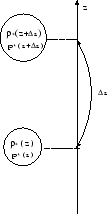If water is heated from the bottom and temperature difference between the top and the bottom exceeds a limit, convection is driven. Water heated from the bottom climbes and cool water on the top descends. This transfers the thermal energy from the bottom to the top. In this section, we describe the condition in which convection is driven.

Consider a hydrostatic balanced atmosphere in which the hydrostatic balance equation is satisfied: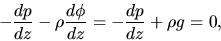(2.78)

where we assumed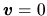in equation (2.2) and the gravity is working downwards in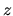-direction (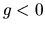) The pressure and density of the atmosphere are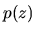and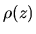. We consider a gas element (hatched region in Fig.2.4), whose density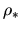and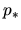are equal to those of the atmosphereandas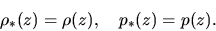(2.79)

Further, we assume this gas element to move fromto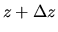adiabatically, that is,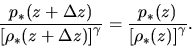(2.80)

Pressure balance is required between the pressures of the gas element at,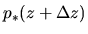, and the atmosphere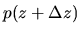. If the density of the gas element at,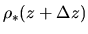, is smaller than that of the atmosphere, the gravity force in equation (2.78) is weaker than the pressure force and the element keeps climbing. Thus the condition for the convective instability is written as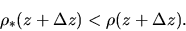(2.81)

Using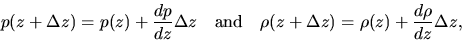(2.82)

we can rewrite equation (2.81) into the relation in the variables of the atmosphere (variables without *) as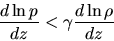(2.83)

or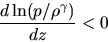(2.84)

This means the specific entropy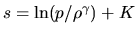decreases upwardly. Thus, if we consider the adiabatic process, the atmosphere in which a specific entropy decreases upwardly is unstable for the convection.

Problem
Obtain equation (2.83) from equation (2.81) using equation (2.82).Next: Super- and Subsonic Flow Up: Physical Background Previous: Rotating Disk   Contents
Kohji Tomisaka 2007-07-08